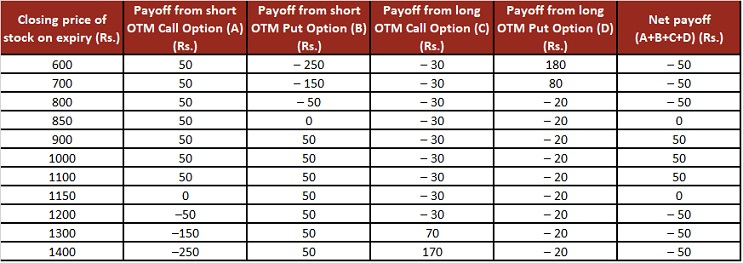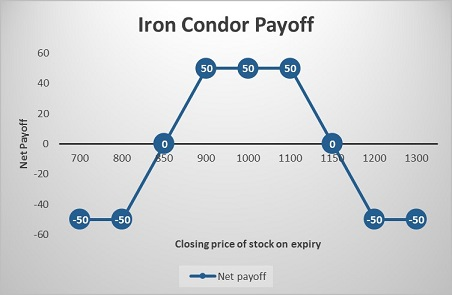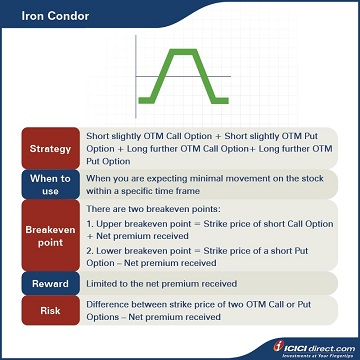With Abhinav knowledge now constantly growing, Simran asks him to think of other strategies that might also work in the rangebound market. Abhinav then suggests the Iron Condor strategy.

## Iron Condor

The Iron Condor strategy is most profitable when the underlying stock or index doesn’t move much and remains in a narrow range. This fell in line with Simran’s market outlook.

The Iron Condor strategy is a four-part Options strategy that comprises two Call Options and two Put Options.

• This strategy involves the selling of Call and Put Options that are slightly OTM and buying further OTM Call and Put Options.
• Buying OTM Call and Put limits your downside risk even if the market moves significantly in any direction.
• By selling slight OTM Calls and OTM Puts, the upside is also limited.

Strategy: Short slightly OTM Call Option (Leg 1) + Short slightly OTM Put Option (Leg 2) + Long further OTM Call Option (Leg 3) + Long further OTM Put Option (Leg 4)

When to use: When you are expecting minimal movement of the stock within a specific time frame

Breakeven: There are two breakeven points:

1. Upper breakeven point = Strike price of short Call Option + Net premium received

2. Lower breakeven point = Strike price of a short Put Option – Net premium received

Maximum risk: Difference between the strike price of two OTM Call or Put Options – Net premium received

Let’s understand the Iron Condor strategy with an example:

Assume that the spot price of PQR Ltd. is Rs. 1,000. Abhinav suggests selling PQR Ltd.’s slightly OTM Call of strike price Rs. 1,100 at Rs. 50 and slightly OTM Put Option of strike price Rs. 900 at Rs. 50. He also suggests buying an OTM Call of strike price of Rs. 1,200 at Rs. 30 and OTM Put of the strike price of Rs. 800 at Rs. 20 to protect from a large move in any direction.

He receives a total premium of Rs. 50 + Rs. 50 = Rs. 100, and pays a total premium of Rs. 50, so the net credit is Rs. 50. This will be the maximum profit per stock.

The difference between Call/Put strike price is 100 and the net premium received is Rs. 50, so the maximum loss will be Rs. 100 – Rs. 50 = Rs. 50.

Upper breakeven point = Rs. 1100 + Rs. 50 = Rs. 1,150

Lower breakeven point = Rs. 900 – Rs. 50 = Rs. 850

### Let’s look at the cash flow in various scenarios:Let us understand the payoff in various scenarios. It will give you a fair idea of how we have arrived at the above values.

### If the stock closes at Rs.700 on expiry: Leg 1 and leg 3 expires OTM, while leg 2 and leg 4 expires ITM

Leg 1: Premium received on the short OTM Call Option of strike price Rs. 1100 = Rs. 50

Premium paid on short OTM Call Option of strike price Rs. 1100 at expiry = Max {0, (Spot price – Strike price)} = Max {0, (700 – 1100)} = Max (0, – 400) = 0

So, the payoff from the short OTM Call Option = Premium received – Premium paid = 50 – 0 = Rs. 50

Leg 2: Premium received on the short OTM Put Option of strike price Rs.900 = Rs. 50

Premium paid on the short OTM Put Option of strike price Rs. 900 at expiry = Max {0, (Strike price – Spot price)} = Max {0, (900 – 700)} = Max (0, 200) = Rs. 200

So, the Payoff from the short OTM Put Option = Premium received – Premium paid = 50 – 200 = – Rs. 150

Leg 3: Premium paid on the long OTM Call Options of strike price Rs. 1200 = Rs. 30

Premium received on the long OTM Call Options of strike price Rs. 1200 = Max {0, (Spot price – Strike price)} = Max {0, (700 – 1200)} = Max (0, – 500) = 0

So, the payoff from the long OTM Call Options = Premium received – Premium paid = 0 – 30 = – Rs. 30

Leg 4: Premium paid on the long OTM Put Options of strike price Rs. 800 = Rs. 20

Premium received on the long OTM Put Options of strike price Rs. 800 = Max {0, (Strike price – Spot price)} = Max {0, (800 – 700)} = Max (0, 100) = Rs. 100

So, payoff from the long OTM Call Options = Premium received – Premium paid = 100 – 20 = Rs. 80

Net Payoff = Payoff from short OTM Call Option + Payoff from short OTM Put Option + Payoff from long OTM Call Option + Payoff from long PTM Put Option = 50 + (– 150) + (– 30) + 80 = – Rs. 50

### If the stock closes at Rs. 1000 on expiry: All four legs expire OTM

Leg 1: Premium received on the short OTM Call Option of strike price Rs. 1100 = Rs. 50

Premium paid on short OTM Call Option of strike price Rs. 1100 at expiry = Max {0, (Spot price – Strike price)} = Max {0, (1000 – 1100)} = Max (0, – 100) = 0

So, the payoff from the short OTM Call Option = Premium received – Premium paid = 50 – 0 = Rs. 50

Leg 2: Premium received on the short OTM Put Option of strike price Rs.900 = Rs. 50

Premium paid on the short OTM Put Option of strike price Rs. 900 at expiry = Max {0, (Strike price – Spot price)} = Max {0, (900 – 1000)} = Max (0, – 100) = 0

So, the Payoff from the short OTM Put Option = Premium received – Premium paid = 50 – 0 = Rs. 50

Leg 3: Premium paid on the long OTM Call Options of strike price Rs. 1200 = Rs. 30

Premium received on the long OTM Call Options of strike price Rs. 1200 = Max {0, (Spot price – Strike price)} = Max {0, (100 – 1200)} = Max (0, – 200) = 0

So, the payoff from the long OTM Call Options = Premium received – Premium paid = 0 – 30 = – Rs. 30

Leg 4: Premium paid on the long OTM Put Options of strike price Rs. 800 = Rs. 20

Premium received on the long OTM Put Options of strike price Rs. 800 = Max {0, (Strike price –  Spot price)} = Max {0, (800 – 1000)} = Max (0, – 200) = 0

So, the payoff from the long OTM Call Options = Premium received – Premium paid = 0 – 20 = – Rs. 20

Net Payoff = Payoff from short OTM Call Option + Payoff from short OTM Put Option + Payoff from long OTM Call Option + Payoff from long PTM Put Option = 50 + 50 + (– 30) + (– 20) = Rs. 50

### If the stock closes at Rs. 1300 on expiry: Leg 1 and leg 3 expires ITM while leg 2 and leg 4 expires OTM

Leg 1: Premium received on the short OTM Call Option of strike price Rs. 1100 = Rs. 50

Premium paid on the short OTM Call Option of strike price Rs. 1100 at expiry = Max {0, (Spot price – Strike price)} = Max {0, (1300 – 1100)} = Max (0, 200) = Rs. 200

So, the payoff from the short OTM Call Option = Premium received – Premium paid = 50 – 200 = – Rs. 150

Leg 2: Premium received on the short OTM Put Option of strike price Rs.900 = Rs. 50

Premium paid on the short OTM Put Option of strike price Rs. 900 at expiry = Max {0, (Strike price – Spot price)} = Max {0, (900 – 1300)} = Max (0, – 400) = 0

So, the Payoff from the short OTM Put Option = Premium received – Premium paid = 50 – 0 = Rs. 50

Leg 3: Premium paid on the long OTM Call Options of strike price Rs. 1200 = Rs. 30

Premium received on the long OTM Call Options of strike price Rs. 1200 = Max {0, (Spot price –  Strike price)} = Max {0, (1300 – 1200)} = Max (0, 100) = Rs. 100

So, the payoff from the long OTM Call Options = Premium received – Premium paid = 100 – 30 = Rs. 70

Leg 4: Premium paid on the long OTM Put Options of strike price Rs. 800 = Rs. 20

Premium received on the long OTM Put Options of strike price Rs. 800 = Max {0, (Strike price – Spot price)} = Max {0, (800 – 1300)} = Max (0, – 500) = 0

So, the payoff from the long OTM Call Options = Premium received – Premium paid = 0 – 20 = – Rs. 20

Net Payoff = Payoff from short OTM Call Option + Payoff from short OTM Put Option + Payoff from long OTM Call Option + Payoff from long PTM Put Option = (– 150) + 50 + 70 + (– 20) = – Rs. 50Did you know?  The iron condor is an improvised version of the short strangle. Can you think of how? Because it protects your downside risk too.

## Summary• The Iron Condor strategy is a four-part Options strategy that comprises two Call Options and two Put Options.  It involves the selling of Call and Put Options that are slightly OTM and buying further OTM Call and Put Options.
• You use this strategy when you are expecting minimal movement on the stock within a specific time frame
• Breakeven: There are two breakeven points:
• Upper breakeven point = Strike price of short Call Option + Net premium received
• Lower breakeven point = Strike price of a short Put Option – Net premium received